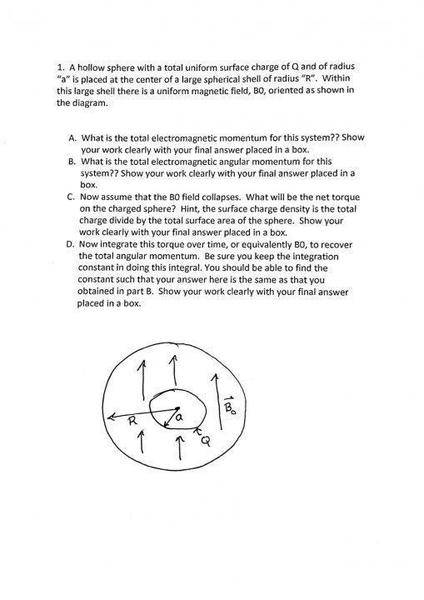# Hollow Sphere with Uniform Surface charge

• woprxcpe1704tks

## Homework Statement[/B]

## Homework Equations

Poynting's Theorem S = 1/μ0 (E x B)
Momentum = p = μ0ε0 ∫ S dτ

## The Attempt at a Solution

My strategy was to treat the hollow sphere as a point charge (by Gauss' Law), so E = 1/4πε0 Q/a2. I believe the magnetic field would be B = 2/3μ0M (where M is the magnetic dipole moment per unit volume). For part A, would this be the correct total electromagnetic momentum if I used the formula stated above and the relevant E and B fields. Also, do I have the E and B field formula's correct?

## Homework Statement

View attachment 95437 [/B]

## Homework Equations

Poynting's Theorem S = 1/μ0 (E x B)
Momentum = p = μ0ε0 ∫ S dτ

## The Attempt at a Solution

My strategy was to treat the hollow sphere as a point charge (by Gauss' Law), so E = 1/4πε0 Q/a2. I believe the magnetic field would be B = 2/3μ0M (where M is the magnetic dipole moment per unit volume). For part A, would this be the correct total electromagnetic momentum if I used the formula stated above and the relevant E and B fields. Also, do I have the E and B field formula's correct?

The attachment is my current attempt at part A

#### Attachments

• Doc Feb 07, 2016, 11_23.pdf
447.3 KB · Views: 195